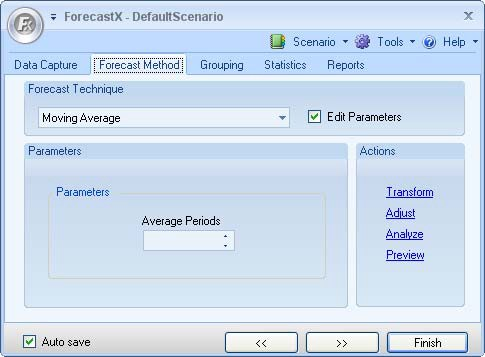# Moving Average

Moving Average is the average of a time series over a specific number of preceding periods. When a new value is added, the last value is removed from the calculation. Therefore, the specific number of preceding periods remains constant.

However, two drawbacks are that Moving Averages do not effectively handle significant trends in data, and all Historical data must be stored to create the Moving Average. In the case of time series data, Moving Average forecasts are often used to eliminate unwanted fluctuations, thereby smoothing the time series. The appropriate number of preceding periods is determined by selecting the number of periods that yields the least amount of error.

The starting value of a Moving Average is where the calculation begins. The start value uses 0 as its first value. If you would like to start at the 4th observation, the start value would be 3. The number of observations to include in the average calculation is called the History. For example, if you want to start at the 5th observation and perform a moving average with 3 Historical Observations, the start value would be 4 because the count starts at 0. The Moving Average calculation would include the 3rd, 4th, and 5th observations.

To use the Moving Average forecasting technique:

1. Click on the Forecast Method tab.
2. In the Forecast Technique area, scroll through the list of methods and select Moving Average. The Moving Average Forecasting technique displays.3. Select Edit parameters to activate Moving Average’s parameters.
4. In the Parameters area, type in the number of Average Periods.
5. Click Finish.